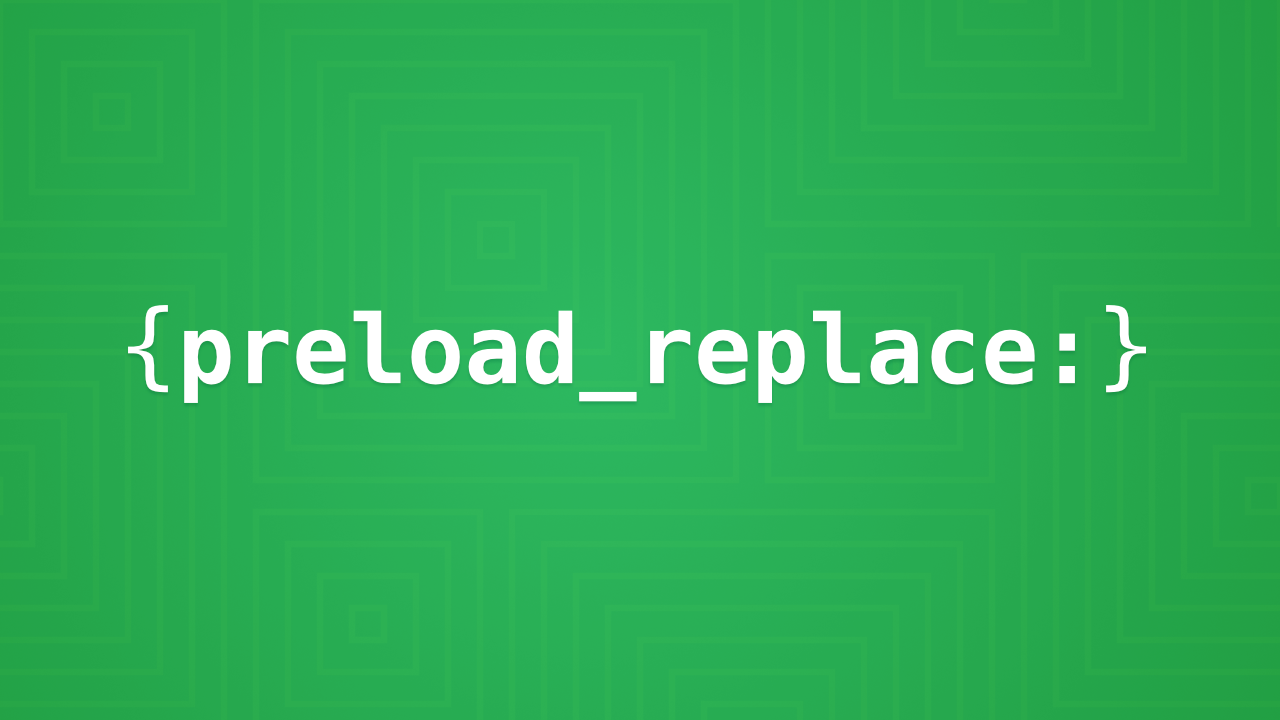Did you know that ExpressionEngine’s preload replace tags support prefixes? Preload replace variables are pretty straight forward. You set a new variable and give it a text value, then later in the template you can access that value. For example if you create a new preload replace variable `{preload_replace:foobar="bazz"}` the variable name `{foobar}` has no context, or namespace. So at first glance it may not be apparent where the variable came from. You can add a prefix to all your preload_replace variables, e.g. `{preload_replace:pre:foobar="bazz"}`, and when accessing it later in the template, the syntax will be `{pre:foobar}`.

Using preload replace variables are pretty straight forward, but order does matter in some cases. For example, the following works as you would expect depending on the value of `segment_2` `{pre:variable}` will print either `bar` or `bazz`.

``````{if segment_2 == "foo"}
{if:else}
{/if}
``````

You can also set a default value without the `{if:else}`:

``````{if segment_2 == "foo"}
{/if}

``````

However, you can’t set the default value before the conditional. If `segment_2` is equal to `foo`, `{pre:variable}` will still print `bazz`.

``````{preload_replace:pre:variable="bazz"}

{if segment_2 == "foo"}
{/if}
``````

Order matters because ExpressionEngine’s template parser only parses the first occurrence of a preload replace variable. If the conditional above evaluates true, the second occurrence of the same preload replace variable is ignored. In most programming languages you define the variable with the default value first, then override it based on how a conditional evaluates.

The following is a practical example of using preload replace. In this case the `{segment:child_category}` variable is created with a custom extension, which could contain a string of IDs, such as `677|678|700`, and we are using a regular expression to check if it contains a specific ID.

``````{if segment:child_category ~ "/(677)/"}
{/if}

{special_events_sections category="{segment:child_category}" limit="1"}
<div class="slab slab--flex theme--aqua-haze">
<section class="slab__cell--{if '{pre:full_width}' == 'yes'}100{if:else}66{/if}">
<div class="slab--flex__container">
<div class="cell__content">
<!-- content -->
</div>
</div>
</section>
{if "{pre:full_width}" != "yes"}
<section class="slab__cell--33">
<div class="slab--flex__container">
<div class="cell__content">
<!-- content -->
</div>
</div>
</section>
{/if}
</div>
{/special_events_sections}
``````

You can also use preload replace variables to define parameters for a partial. ExpressionEngine’s template partials do not support embed parameters, e.g. `{embed="group/template" foo="bar"}`, but you can mimic that behavior with a preload replace. The following is a bit of a contrived example, but it illustrates how you can use preload replace to make a partial that can be configured based on how and where it is used.

``````<article class="heading heading--{pre:section}">
</article>
``````

When using that partial you can define the preload replace variables just before the partial:

``````{preload_replace:pre:heading_tag="h2"}